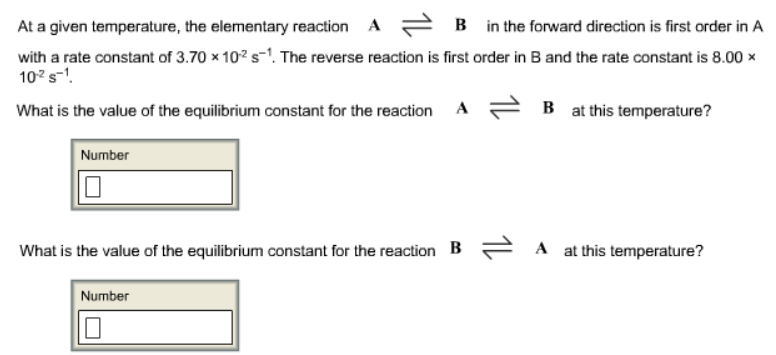Chemistry Practice Problems Equilibrium Expressions Practice Problems Solution: At a given temperature, the elementary reaction A ...

# Solution: At a given temperature, the elementary reaction A ⇌ B in the forward direction is first order in A with a rate constant of 3.70 x 10-2 s-1. The reverse reaction is first order in B and the rate constant is 8.00 x 10-2 s-1. What is the value of the equilibrium constant for the reaction A ⇌ B at this temperature? What is the value of the equilibrium constant for the reaction B ⇌ A at this temperature?

###### Problem

At a given temperature, the elementary reaction A ⇌ B in the forward direction is first order in A with a rate constant of 3.70 x 10-2 s-1. The reverse reaction is first order in B and the rate constant is 8.00 x 10-2 s-1

What is the value of the equilibrium constant for the reaction A ⇌ B at this temperature?

What is the value of the equilibrium constant for the reaction B ⇌ A at this temperature?View Complete Written Solution

Equilibrium Expressions

Equilibrium Expressions

#### Q. Write the equilibrium-constant expression for the reaction2 NO(g) + O2(g) ⇌ 2 NO2(g)in terms of [NO], [O2], and [NO2].

Solved • Mon Aug 13 2018 16:53:24 GMT-0400 (EDT)

Equilibrium Expressions

#### Q. At a certain temperature, a 29.0-L contains holds four gases in equilibrium. Their masses are: 3.5 g SO3, 4.6 g SO2, 24.2 g N2, and 0.98 g N2O. What i...

Solved • Thu Aug 09 2018 13:23:37 GMT-0400 (EDT)

Equilibrium Expressions

#### Q. Calculate the value of Kp for the equation C (s) + CO2 (g) ⇌ 2CO (g) Kp = ? given that at a certain temperature C (s) + 2H2O (g) ⇌ CO2 (g) + 2H2 (g) K...

Solved • Wed Aug 01 2018 14:29:45 GMT-0400 (EDT)

Equilibrium Expressions

#### Q. When Kc is &lt;&lt;1, the equilibrium lies far to the right.a. Trueb. False

Solved • Wed Aug 01 2018 13:26:23 GMT-0400 (EDT)